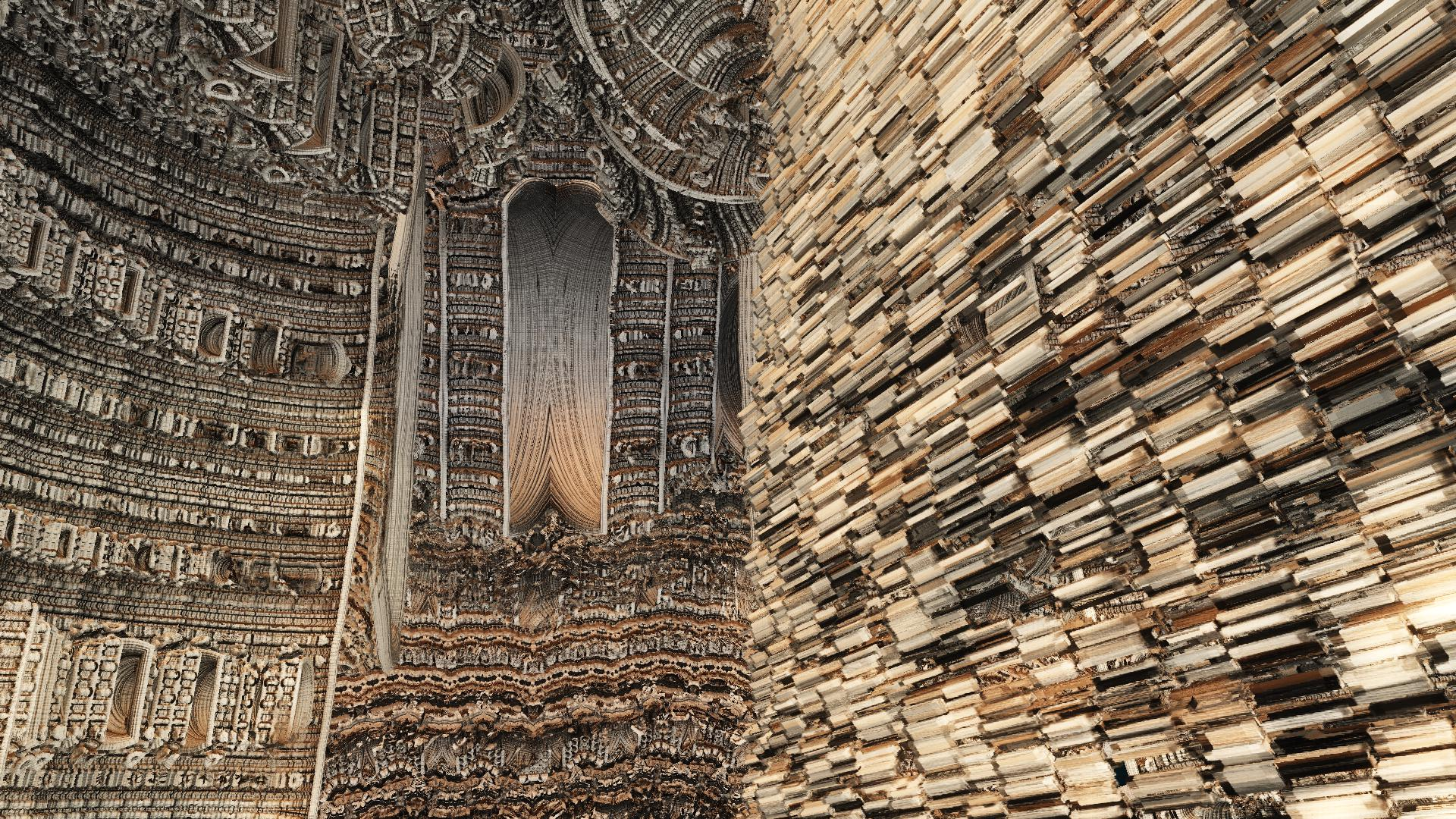News: Follow us on Twitter## The All New FractalForums is now in Public Beta Testing! Visit FractalForums.org and check it out!

PaperbackPrevious Image | Next Image
Description: Paperback
Fragmentarium Kali's Asurf
From Kalis Rotated Asurf Frag ..
As a  big welcome back to kali and a thanks to him for his great Fractal code

Code:
// Output generated from file: C:/Users/USER/Documents/ScOrPiOn2016/remakeasurf.jpg Files/remakeasurf.frag
// Created: Wed Sep 14 16:52:00 2016
// Output generated from file: C:/Users/USER/Documents/Fragmentarium by  SCORPION/explodes2.jpg Files/explodes2.frag
// Created: Mon Sep 12 22:23:37 2016
// Output generated from file: C:/Users/USER/Desktop/Fragmentarium by  SCORPION/Examples/Examples New Raytracer/VCRaytracer/Explosion.frag
// Created: Mon 2. Feb 16:08:28 2015
#define providesInit
#include "MathUtils.frag"
#include "DE-Kn2cr11.frag"

#group Rotation
uniform vec3 RotationCenter; slider[(-1,-1,-1),(0,0,0),(1,1,1)]

#group AmazingSurface
uniform int Iterations;  slider[0,17,300]
uniform int ColorIterations;  slider[0,3,300]
uniform float Scale;  slider[-3,1.5,3.0]
uniform int FoldType; slider[1,1,3]
uniform vec3 PreTranslation; slider[(-5,-5,-5),(0,0,0),(5,5,5)]
uniform vec2 FoldValues; slider[(0,0),(1,1),(5,5)]
uniform bool Julia; checkbox[false]
uniform vec3 JuliaValues; slider[(-5,-5,-5),(-1,-1,-1),(5,5,5)]
uniform vec3 RotVector; slider[(-1,-1,-1),(1,1,1),(1,1,1)]
uniform float RotAngle; slider[-180,0,180]

mat3 rot;

void init() {
rot = rotationMatrix3(normalize(RotVector), RotAngle);
}

//float scale = Scale/MinRad2;//scalar derivative doesn't need to be multiplied with ABS(scale) but with scale instead.
float absScalem1 = max(1.,abs((1.-Scale)/(1+Scale)))*2.;//Size of the mandelbox with scale = - Scale. If IIRC.
float bailout=100.*absScalem1*absScalem1;//julias are usually big things.
//float AbsScaleRaisedTo1mIters = pow(abs(Scale), float(1.-float(Iterations)));

float DEA(vec3 pos) {
vec4 p = vec4(pos,1.);
vec4 c = Julia?vec4(JuliaValues,0.):p; //using vec4 in order to have the add +1 to scalar derivative in case it's not a julia. This is necessary to have a correct DE when not in julia mode.
float r2= dot(p.xyz, p.xyz);
for (int i=0; i<Iterations; i++) {
if (FoldType==1) p.xy=p.xy-abs(p.xy+FoldValues)+abs(p.xy-FoldValues);//I've flipped the sign -> derivative = 1 for x,y large enought. This way, I just multiply the scalar derivative by Scale without taking its abs. value.
if (FoldType==2) p.xy=FoldValues-abs(abs(p.xy)-FoldValues);
if (FoldType==3) p.xy=abs(p.xy+FoldValues);
p.xyz+=PreTranslation;
r2 = dot(p.xyz, p.xyz);
if (i<ColorIterations) orbitTrap = min(orbitTrap, abs(vec4(p.xyz,r2)));
p = p * k + c;
p.xyz*=rot;
r2 = dot(p.xyz, p.xyz); if(r2>bailout) break;//saves some iteration. especially when  Iterations is large.
}
return (length(p.xyz) - absScalem1) / abs(p.w) ;
}

float DE(vec3 p) {
p=p-RotationCenter;
float r=length(p);
float r2=r*r;
float de=DEA(p);
return de;
}

#preset paperbck
FOV = 0.42962
Eye = -0.589376,-4.026,4.51817
Target = -1.93218,-4.05945,3.49053
UpLock = false
FocalPlane = 2.28
Aperture = 0
InFocusAWidth = 0
DofCorrect = true
ApertureNbrSides = 7
ApertureRot = 0
ApStarShaped = false
Gamma = 0.98825
ToneMapping = 5
Exposure = 0.72045
Brightness = 0.907
Contrast = 1.55705
Saturation = 1.9233
GaussianWeight = 0.9069
AntiAliasScale = 0.2528
Bloom = true
BloomIntensity = 0.78644
BloomPow = 5.4899
BloomTaps = 12
BloomStrong = 6.98044
Detail = -2.91221
RefineSteps = 4
FudgeFactor = 1
MaxRaySteps = 615
MaxDistance = 39
Dither = 0.36995
NormalBackStep = 1.8333
DetailAO = -1.00002
coneApertureAO = 0.5
maxIterAO = 20
FudgeAO = 1
AO_ambient = 0.7
AO_camlight = 0
AO_pointlight = 0
AoCorrect = 0
Specular = 0.18391
SpecularExp = 14.545
CamLight = 1,1,1,0.4318
AmbiantLight = 1,1,1,1
Reflection = 1,1,1
ReflectionsNumber = 0
SpotGlow = true
SpotLight = 1,0.682353,0.45098,6.4496
LightPos = 1.7054,5.5504,1.2248
LightSize = 0.15639
LightFallOff = 0.36236
LightGlowExp = 2.6058
perf = false
SSS = false
sss1 = 0.21607
sss2 = 0.11221
BaseColor = 1,1,1
OrbitStrength = 0.28582
X = 0,0,1,0.43356
Y = 1,0.6,0,0.67046
Z = 0.8,0.78,1,0.4909
R = 0.4,0.7,1,0.26292
BackgroundColor = 0.270588,0.780392,1
CycleColors = true
Cycles = 3.85677
EnableFloor = false
FloorNormal = 0,0,1
FloorHeight = 0
FloorColor = 1,1,1
HF_Fallof = 0.1
HF_Const = 0
HF_Intensity = 0
HF_Dir = 0,0,1
HF_Offset = 0
HF_Color = 1,1,1,1
HF_Scatter = 0
HF_Anisotropy = 0,0,0
HF_FogIter = 1
EnCloudsDir = false
Clouds_Dir = 0,0,1
CloudScale = 1
CloudFlatness = 0
CloudTops = 1
CloudBase = -1
CloudDensity = 1
CloudRoughness = 1
CloudContrast = 1
CloudColor = 0.65,0.68,0.7
CloudColor2 = 0.07,0.17,0.24
SunLightColor = 0.7,0.5,0.3
Cloudvar1 = 0.99
Cloudvar2 = 0.99
CloudIter = 5
CloudBgMix = 1
RotationCenter = -0.8205,-0.43862,0.6122
Iterations = 51
ColorIterations = 9
Scale = 1.58064
FoldType = 2
PreTranslation = -1.0457,-0.5288,0.2043
FoldValues = 5,0.7281
Julia = true
JuliaValues = 4.2537,-0.9701,-1.1439
RotVector = 1,-0.16432,0.01644
RotAngle = -19.4076
Up = 0.39431,1.11305,-0.751692
#endpreset

Stats:
Total Favorities: 0 View Who Favorited
Filesize: 751.15kB
Height: 1080 Width: 1920
Discussion Topic: View Topic
Keywords: timemit fragmentarium asurf kali library paperback
Posted by: Tim EmitOctober 30, 2016, 12:01:33 PM

Rating:by 4 members.

 Comments (2)Caleidoscope Fractal FertilizerPosts: 365November 03, 2016, 09:42:14 AMWell, it is probably the index of the library3dickulus Global Moderator Fractal SeniorPosts: 1558November 01, 2016, 03:29:43 AMhmm... I wonder what's in that dusty old volume way in the back